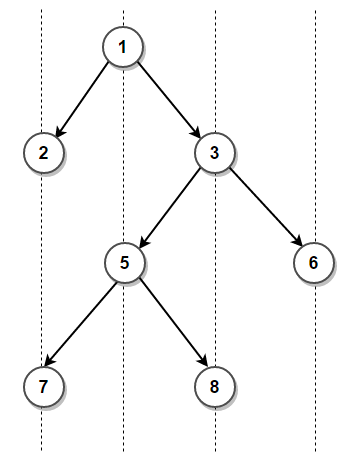# How To Print nodes of a given binary tree in vertical order?

Given a binary tree, print its nodes in vertical order. Assume that the left and right child of a node makes 45 degree angle with the parent.

For example, nodes in vertical order for following binary tree is

2, 7
1, 5
3, 8
6In the previous post, we have seen hash table implementation which takes O(nlog(n)) time. We can improve time complexity of above program to linear by with the help of an auxiliary data structure such as a doubly linked list. The idea here is to store vertical order of the binary tree in a doubly linked list where each node of doubly linked list stores every node corresponding to a vertical line in a binary tree. This post provides an overview of some of the available alternatives to accomplish this using a doubly linked list.

### 1. Using Preorder Traversal

We start by constructing a doubly linked list node which stores all nodes present at vertical line passing through the root node. Then node->prev and node->next will correspond to nodes present at vertical line passing through the left and right child of the root node respectively. The trick is to recursively construct the linked list and add nodes to it as we traverse the tree using pre-order traversal.
The algorithm can be implemented as follows in C++:

Output:

2 7
1 5 9
3 8
10 6

### 2. Using Level Order Traversal

Since above solution uses preorder traversal to traverse the tree, the nodes might not get processed in same order as they appear in the binary tree from top to bottom. For instance, node 10 is printed before node 6 in above solution.
We can perform level order traversal to ensure the nodes are processed in same order as they appear in the binary tree. The idea remains the same as previous approach except we traverse the binary tree using level order traversal instead of the pre-order traversal. This is demonstrated below in C++:

Output:

2 7
1 5 9
3 8
6 10

The time complexity of both above solutions is O(n) and auxiliary space used is O(n) for doubly linked list.